5.3 Mean, Median and Mode for ungrouped data:

You must have heard your teachers saying that the average number of students in a class in their school is 45. What does it mean?

Normally a school has several sections for a particular standard (Ex: Sections A, B and C in 8th standard). Most of the times,all the sections in a school will not have same number of students.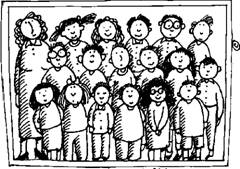Class A                                               Class B                                       Class C

5.3 Example 1: In the above picture ‘A’ section has 47, ‘B’ section has 42 and ‘C’ section has 46 students. If you add the number of students of all these sections you will get 135(47+42+46). If you divide this by 3 (number of sections), you get 45(=135/3). This number (=45) is what teachers mean by average number of students in a class in their school. Similarly you can arrive at the average number of students in a class for the entire school (considering all sections of all standards). If there is only one section and the section has 40 students, then average and class strength are one and the same.

We have seen in statistics that the individual figures (47,42,46) are called scores and the number 3 (no of sections in this case ) is called Number of scores.

5.3 Example 2: You must have heard your parents saying that you have scored an average of 68 marks in an examination. Since you have several subjects, it is difficult to remember the marks in each of the subjects. Because, it is easy to remember one number, people express the marks as average. Let the marks scored be: English: 65, Hindi: 60, Social Science: 65, General science: 70, Mathematics: 80.If you add all the marks, you get 340. Since there are 5 subjects we divide the total marks by this number to get 68. This is the average marks you have got. In this method you must have observed that the average does not reflect your highest marks(got in mathematics) or lowest marks(got in Hindi).

Note: As it can be seen from the above example, expressing average figure could be misleading in some cases. But it is very useful in many cases particularly when we have large number of scores (Ex: average rain fall in a place, average height of students in a class, etc).

5.3 Example 3: Let your favorite cricketer score the following runs in few one day matches

27,45,40,18,80,55, 47,105,46, 40,47. What is his average?

Workings:

Total runs scored = 27+45+40+18+80+55+ 47+105+46+40+47=550

Number of matches played = 11

Average Runs per match = 550/11=50

Average can be calculated by applying the following formula:

Definitions:

‘Mean’ = Sum of all scores/Number of Scores

Let  x1,x2,x3,x4   ….xn be scores( thus there are ‘n’ number of scores)

Then Average = (x1+x2+x3+x4   ….+xn)/n

Mean = (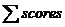)/Number of scores

The symbol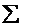is pronounced as sigma and is used to represent the sum of numbers.

The mean is also called average’ or ‘Arithmetic mean’.

Let us learn few more concepts in statistics.

Let us arrange the runs of Example 5.3.3 in ascending order. Then the runs are

18,27,40,40,45,46,47,47,55,80,105.

Middle figure (6th out of 11) in this arrangement is 46 and is called Median.

The middle most figure in an orderly distribution of scores is called ‘median’.

It is interesting to note that in this Cricketer’s case, his Mean (Average) (50) and Median (46) are very close to each other.

Let us find the most occurrences of same number of runs scored, in case of this cricketer. We find that he scored 40 runs and 47 runs two times each. They are called Mode.

The Mode’ is the most often repeated score in a given set of scores.

In the above example the number of matches was 11.  Since 11 is  an odd number, it is  easy to find Median (middle figure). What if we have even  number of scores ?

5.3 Example 4: Let us take the case of temperatures recorded (in centigrade) for 10 days at your place.

Let they be   250 C, 300 C, 310 C,340 C,320 C,310 C,300 C,280 C,300 C,310 C.

Working:

When we arrange them in ascending order, we get

250C,280C,300 C,300 C,300 C,310 C,310 C,310 C,320 C,340 C.

Let us tabulate them in a table:

 Scores (x) Occurrences (frequency)(f) fx=x*f 250C 1 25 280C 1 28 300C 3 90 310C 3 93 320C 1 32 340C 1 34 Total() 10 302

Average For ungrouped data (when scores and frequency are given) can also be calculated by the formula

Average (mean) = (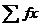)/ (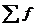)= 302/10 = 30.20C

Median is (30+31)/2 =30.50C (Average of 5th and 6th term as there are even number of scores which is 10)

Modes are 300C and 310C both of which occur three times which is the most number of occurrences.

Note: In this Example there is not much difference between Average (30.20C),Median (30.50C) and Modes(300C,310C). When we have large number of data (scores) we may notice Average, Median, and Mode being nearer to each other.

5.3 Problem 1: Mean of 9 observations was found to be 35. Later on it was found that an observation 81 was misread as 18. Find the correct mean of observations.

Solution:

Mean of 9 observations = 35Sum of all observations = 35*9 = 315

In the observations 81 was misread as 18

The correct sum of all observations = 315-18+81 = 378The correct mean = 378/9 = 42

5.3 Summary of learning

 No Points to remember 1 Mean= (Sum of all scores)/Number of scores 2 The middle most figure in an orderly distribution of scores is called median 3 Mode is the most often repeated score in a given set of scores

5.3.2 Assumed mean method for calculation of mean.

This method is very useful when data and their frequencies are very large. In this method we assume one of the data to be mean and find the deviation from that number and hence this method is called  ‘assumed mean method’.

Let us take the  example worked out earlier (5.3 Example 4) to illustrate this method.

5.3 Example 4: Let us take the case of temperatures recorded (in centigrade) for 10 days at your place.

Let they be 250C, 300C, 310C, 340C, 320C, 310C, 300C, 280C, 300C, 310C.

Let  30  be the assumed mean (any score can be assumed to be the mean but we normally take the score which is in the middle part of the distribution as assumed mean)

The Deviation D (D = Score- Assumed mean) is calculated for each of the score.

Then Average (mean) = A + (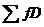)/Number of scores

 Scores (x) Frequency(f) Deviation D= A-M fD = f*D 250C 1 -5(=25-30) -5 280C 1 -2(=28-30) -2 300C=A 3 0(=30-30) 0 310C 3 1(=31-30) 3 320C 1 2(=32-30) 2 340C 1 4(=34-30) 4 Total() 10 2

Average (Mean) =  A +()/Number of scores = 30+ (2/10) = 30.2

Is this not the same mean value which we got earlier?

This method is less time consuming and hence less chances of one making mistakes.